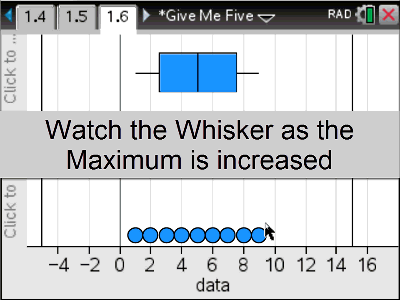### Mathematics lessons for IB® Diploma Programme

Analysis and Approaches | Statistics and Probability## Give Me Five

### Objectives

This activity involves describing the centre and spread of a univariate data set by way of a five-figure summary and visually by a box and whisker plot.

### Vocabulary

• Minimum
• Q1 = Quartile 1
• Median ( Q2 )
• Q3 = Quartile 3
• Maximum
• Outlier
• Upper Fence
• Lower Fence
• Left / Right Skew
• Box and Whisker Plot